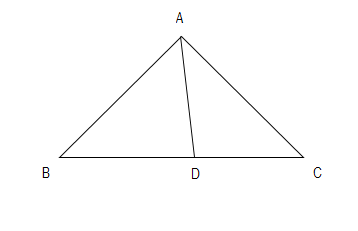QUESTION

# In $\Delta ABC,$ the internal bisector AD of $\angle A$ meets the side BC at D. If BD=2.5cm, AB=5cm and AC=4.2cm, then find DC.

Hint: Start by drawing a neat diagram. Then apply the theorem that the internal angle bisector of a triangle divides the opposite side of the triangle in the ratio of its other two sides.Now it is given in the question that AD is the angle bisector of $\angle BAC$. So, we can say $\angle BAD=\angle CAD$ .
Also, it is given in the question that AB=5cm, AC=4.2cm and BD=2.5cm.
Now, as we know that the angle bisector of the internal angle of the triangle divides the opposite sides in the ratio of the other two sides of the triangle. So, we can represent this mathematically as:
$\dfrac{BD}{DC}=\dfrac{AB}{AC}$
Now we will put the values given in the question. On doing so, we get
$\dfrac{2.5}{DC}=\dfrac{5}{4.2}$
On cross-multiplication, we get
$2.5\times 4.2=5\times DC$
Now we will take 5 to the other side of the equation. On doing so, our equation becomes:
$\dfrac{2.5\times 4.2}{5}=DC$
$\Rightarrow DC=2.1cm$
Therefore, we can conclude that the length of DC is 2.1cm.

Note: The key to such questions is the diagram. If you get the diagram correct, then there is a high possibility that you would reach an answer provided you have the basic knowledge of regularly used theorems, as we saw in the above question. Also, the general mistake that a student makes is getting confused between the medians and the angle bisectors leading to errors, so be careful while using the properties of medians and angle bisectors.